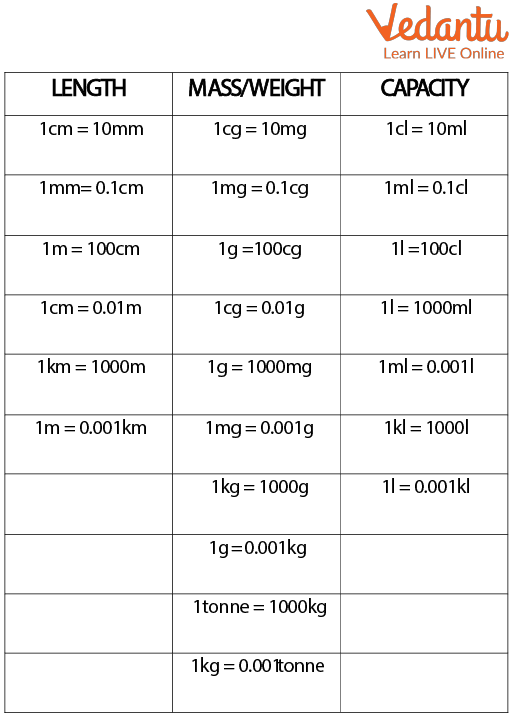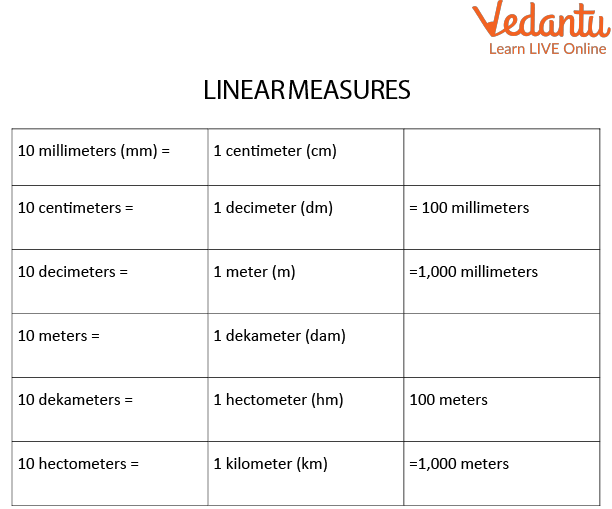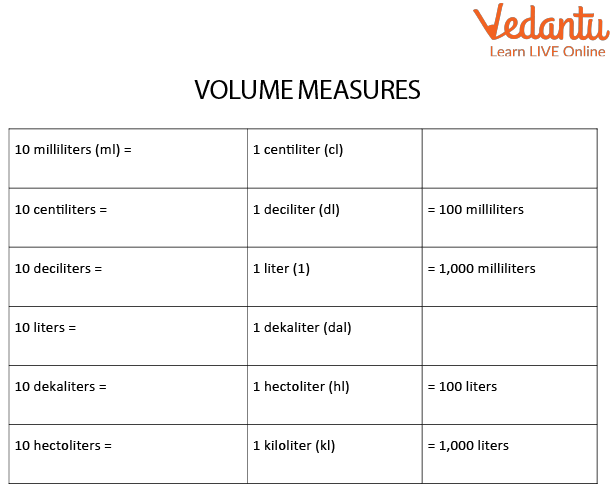Courses
Courses for Kids
Free study material
Offline Centres
More

# Metric MeasuresLast updated date: 01st Dec 2023
Total views: 99k
Views today: 1.99k## Introduction to Metric Measures

The most common method for determining height, distance, and weight is the metric system of measurement. By definition, the metric system of measurement in mathematics is the set of standard units defined to measure length, weight, area, and capacity. It is based on the decimal system as it includes numbers in powers of 10. The base units of length (distance), capacity (volume), and weight (mass) in the metric system are the metre, litre, and gramme, respectively. Measurements for length, width, and height are made using millimetres (mm), decimeters (dm), centimetres (cm), metres (m), and kilometres (km).

## Metric System of Weights and Measures Used in the Unit

For measuring length, mass, area, and capacity, metric units come in a variety of shapes and sizes. The metric units for measuring length include millimetres, centimetres, metres, and kilometres. The units used to measure weight are grammes and kilograms. To grasp all the metric system units used for various applications, look at the table below which shows the metric system of weights and measures used in the unit as:

 Purpose Metric Units Abbreviated As Measurement of length Millimetres mm Centimetres cm Metres m Kilometres km Mass/weight measurement Milligrams mg Centigrams cg Grams g Kilograms kg Tonne t Measurement of area Square centimetres Sq. cm Square metres Sq. m Square kilometres Sq. km Hectare $(10,000$ square metres) ha Capacity Measurement Milliliters ml Centiliters cl Litres l Kiloliters kl

## Conversion of Metric Measures

Converting from one metric system to another is known as the conversion of metric measures. The metric conversion ratio is a ratio that is equivalent to 1 in unit measurements. Unit factors are another name for it. The following list includes some of the most popular metric system conversion formulas:

• Multiply 1000 to change km to m.

• Multiply 100 to convert from m to cm.

• Multiply 10 to convert 10 cm to mm.

• Multiply 1000 to convert kilogrammes to grammes.

• Multiply 1000 to convert grammes to milligrammes.

• Divide 1000 to convert from litres to kiloliters.

• Divide 1000 to convert millilitres to litres.

### Chart for Metric Measures

The conversion formulas for the various metric units are contained in the chart of metric units. By examining its multiplication factor, you can rapidly convert one unit to another. For instance, you can see from the metric measures chart that 1 metre equals 100 centimetres. Let's look at the diagram of the metric system below:Conversions

### Metric Measures Table

We have linear, area, volume, and cubic measures as follows:Linear measuresVolume measures

Table for Cubic Measures

 1,000 cubic millimetres $\left(\mathrm{mm}^3\right)=$ $1 \mathrm{cu}$ centimetre $\left(\mathrm{cm}^3\right)$ 1,000 cubic centimetres $=$ 1 cu decimeter $\left(\mathrm{dm}^3\right)$ $=1,000,000$ cu millimetres 1,000 cubic decimeters $=$ 1 cu metre $\left(m^3\right)$ 1,000 cubic decimeters $=$ = 1 stere 1,000 cubic decimeters $=$ $=1,000,000$ cu centimetres 1,000 cubic decimeters $=$ $=1,000,000,000$ cu millimetres

## What do the Metric Measures Indicate?

Metric measure indicates the base units of length (distance), capacity (volume), and weight (mass) in the metric system as metre, litre, and gram, respectively. We utilise units that are derived from metric units to measure smaller or greater quantities.

## Solved Examples

Q 1. Conversion of 500 metres to kilometres.

Ans: We know that

$1 m=\dfrac{1}{1000} \mathrm{~km}$

For 500 metres we have

$500 m=\dfrac{500}{1000} k m$

$500 m=\dfrac{1}{2} k m=0.5 \mathrm{~km}$

Thus, 500 metres is half a kilometre.

Q 2. In an aquarium, Paul spotted a 600 cm long enormous fish. Calculate its length in millimetres.

Ans. The abbreviations for two of the length measurement units of the metric system are centimetres and millimetres or $\mathrm{cm}, \mathrm{mm}$ respectively.

Since there are $10 \mathrm{~mm}$ in 1 $\mathrm{cm}$, multiply the provided amount by 10 to convert from centimetres to millimetres.

$1 \mathrm{~cm}=10 \mathrm{~mm}$

$600 \mathrm{~cm}=600 \times 10 \mathrm{~mm}$

$600 \mathrm{~cm}=6000 \mathrm{~mm}$

Q 3. Convert 3 km to m.

Ans: To convert from kilo to hecto, hecto to deca and deca to metre, we need to multiply it by 10.

So, we multiply by $10^{3}=1000$

$3 \mathrm{~km}=3 \times 1,000=3,000 \mathrm{~m}$.

Q 4. Convert 20 mm to cm.

Ans: To convert from milli to centi, we need to divide it by 10.

So, we divide by $10 .$

$20 \mathrm{~mm}=20 \div 10=2 \mathrm{~cm}$

Q 5. A wireless router supports a range of up to 4,572 cm indoors. Calculate that length in metres.

Ans: Since, 100 cm equals to 1 m. Therefore, to find for 4,572cm; we need to divide it by 100 to convert from cm to m.

Therefore, $\dfrac{4,572}{100}$

= 45.72 m

So, a wireless router supports a range of up to 45.72 metres.

## Practice Questions

Q 1. Conversion of 356 centimetres to metres.

Ans: 3.56 metres

Q 2. Rahul finds a garden having a length as 500 cm. Calculate its length in millimetres.

Ans: 5000 mm

Q 3. Calculate a woman’s body mass of 53 kg in grams.

Ans: 53000 Grams

Q 4. How many metres are in 5.0 cm?

Ans: 0.050 cm

## Summary

The metric system of measurement in mathematics is the set of standard units defined to measure length, weight, and capacity. The length/distance conversion chart gives the basic unit conversions related to length in a simple and easy form. The area Conversion chart gives the basic unit conversions related to the area. The area is the space occupied by a two-dimensional shape or figure. The area is measured in square units. The volume conversion chart gives the basic unit conversions related to volume. The term capacity or volume is used for measuring the space occupied by the object. Volume is the space enclosed or occupied by any three-dimensional object or solid shape. It has length, width, and height. It is measured in cubic units. In the end we have added some solved examples, on conversion of units. This will help in understanding and practising the questions.

## FAQs on Metric Measures

1. What is the metric scale smallest to biggest order?

The most common metric length units are listed below, from smallest to largest.

• The smallest measurement is a millimetre.

• The next smallest measurement is a centimetre. Longer lengths are expressed in kilometres.

• As a millimetre is the smallest unit which is beneficial for measuring small things, a centimetre is again the smaller unit and metres are greater than three feet whereas a kilometre is useful for long distances.

2. How should metric measures be expressed?

For instance, $7 m, 31.4 m, 37^{\circ} \mathrm{C}$. It is not essential to hyphenate a metric value when it is used as a one-thought modifier before a noun. If a hyphen is required, it must be placed between the numeral and the quantity in the name of the metric quantity. The metre, the SI's preferred unit of length, is somewhat longer than a yard. A number and a unit must be used together to express a quantity. If your length is 2.4 m, you would just say "2.4 m" to represent it.

3. Mention any two advantages of the metric system over traditional units.

Without the metric system, we'd have a different International System of Units, the metric system is important because 1 mm is 0.1cm, 1 cm is 0.01m, with the imperial system the conversion is tedious. The metric units are accurate whereas the traditional units were not uniform. The metric system is accepted globally whereas the traditional units had different values at different places.